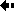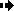## Rate of evolutionThe rate of evolution is a measurement of the change in an evolutionary lineage over time.

The method for measuring the rate of evolution comes from the famous work by MacFadden on horse teeth.

The rate of evolution is measured as followed:

Suppose that a character has been measured at two times, t1 and t2; t1 and t2 are expressed as times before the present in millions of years.

The time interval between the two samples can be written as:

Dt = t1 - t2,

which is 1 million years if t1 = 15.2 and t2 = 14.2 .

The average value of the character is defined as x1 in the earlier sample and x2 in the later sample; we then take natural logarithms of x1 and x2 (the natural logarithm is the log to base e where e = 2.718, and it is symbolized by log).

The evolutionary rate (r) then is

r = (log(x2) - log(x1)) / Dt.

Haldane defined a 'darwin' as a unit to measure evolutionary rates; one darwin is a change in the character by a factor of e in one million years.

The formula above for r gives the rate in darwins provided that the time interval is in millions of years.Previous Next